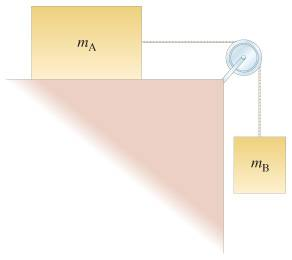# Acceleration of a triple Atwood Machine

Calpalned

## Homework Statement

There is no friction at all and this is a pulley system. The pulleys and string are mass-less. The numbers correspond to the block weight (ex: 3 = 3kg).

F = ma

## The Attempt at a Solution

From my solutions guide, it seems that the acceleration of block 3 is not equal to the other two blocks. I do not understand this. They are all connected by the same string and therefore are influenced by the same force of tension.

Last edited by a moderator:

Homework Helper

## Homework Statement

There is no friction at all and this is a pulley system. The pulleys and string are mass-less. The numbers correspond to the block weight (ex: 3 = 3kg).

F = ma

## The Attempt at a Solution

From my solutions guide, it seems that the acceleration of block 3 is not equal to the other two blocks. I do not understand this. They are all connected by the same string and therefore are influenced by the same force of tension.

What forces act on the hanging block?

The length of the rope is unchanged, but it is shared among the two horizontal pieces and the two (equal) vertical pieces. They do not need to change with the same rate. Imagine that block 2 is very-very-very heavy, so it is practically stationary....

Calpalned
I see... so the blocks don't have the same acceleration?

Calpalned
If that's the case why do the two blocks below have the same acceleration? Here the blocks have different masses and are connected by the same string. The pulley system in my first post also only uses one string, but yet acceleration varies?Homework Helper
If that's the case why do the two blocks below have the same acceleration? Here the blocks have different masses and are connected by the same string. The pulley system in my first post also only uses one string, but yet acceleration varies?
It's a different situation.
In post #1, if you hold one of the blocks still, the other two blocks still have freedom to move. But in that picture in post #4, if you hold one of the blocks still, the other block cannot move (without the rope becoming slack).

Can you see how if block 3 in post #1 was fixed, block 1 and 2 can still move left or right? This is why they don't have to have the same acceleration; there is more freedom in the system.
You can see block 1 and 2 have the same force of tension acting on them (right?) so they will in fact have different accelerations.